## Eigenvector

A right eigenvector satisfies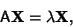(1)

whereis a column Vector. The right Eigenvalues therefore satisfy(2)

A left eigenvector satisfies(3)

whereis a row Vector, so(4)(5)

where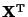is the transpose of. The left Eigenvalues satisfy(6)

(since) whereis the Determinant of A. But this is the same equation satisfied by the right Eigenvalues, so the left and right Eigenvalues are the same. Letbe a Matrix formed by the columns of the right eigenvectors and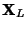be a Matrix formed by the rows of the left eigenvectors. Let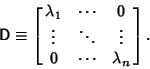(7)

Then(8)(9)

so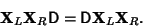(10)

But this equation is of the form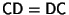where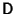is a Diagonal Matrix, so it must be true thatis also diagonal. In particular, if A is a Symmetric Matrix, then the left and right eigenvectors are transposes of each other. If A is a Self-Adjoint Matrix, then the left and right eigenvectors are conjugate Hermitian Matrices.

Given a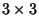Matrix A with eigenvectors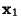,, andand corresponding Eigenvalues,, and, then an arbitrary Vectorcan be written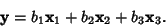(11)

Applying the Matrix A,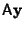(12)

so(13)

If, it therefore follows that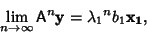(14)

so repeated application of the matrix to an arbitrary vector results in a vector proportional to the Eigenvector having the largest Eigenvalue.

Arfken, G. Eigenvectors, Eigenvalues.'' §4.7 in Mathematical Methods for Physicists, 3rd ed. Orlando, FL: Academic Press, pp. 229-237, 1985.
Press, W. H.; Flannery, B. P.; Teukolsky, S. A.; and Vetterling, W. T. Eigensystems.'' Ch. 11 in Numerical Recipes in FORTRAN: The Art of Scientific Computing, 2nd ed. Cambridge, England: Cambridge University Press, pp. 449-489, 1992.